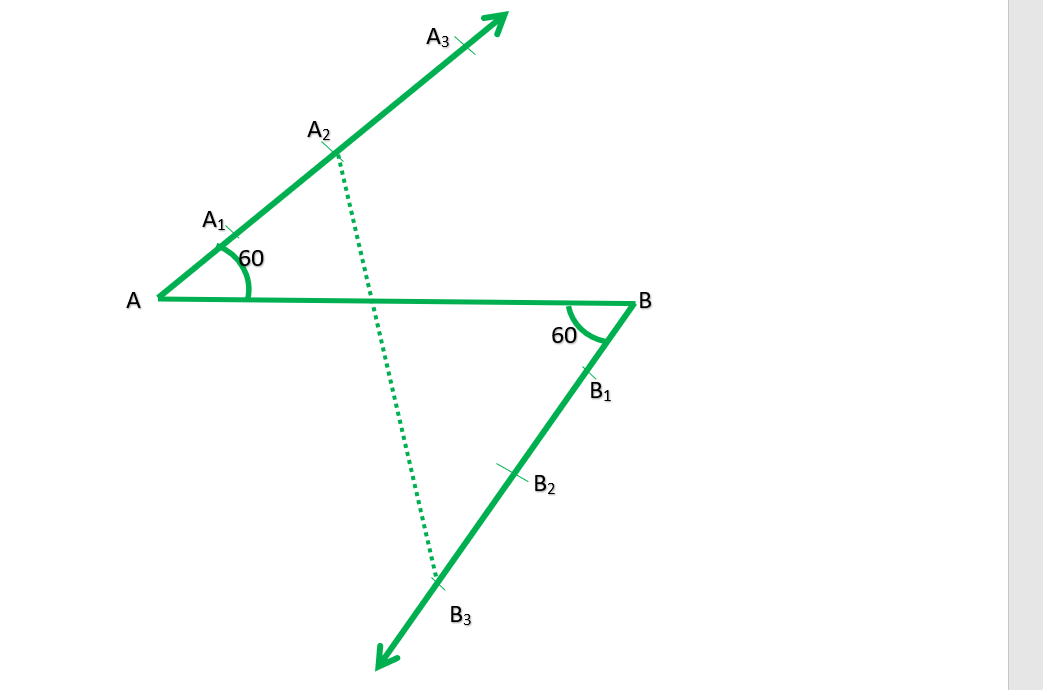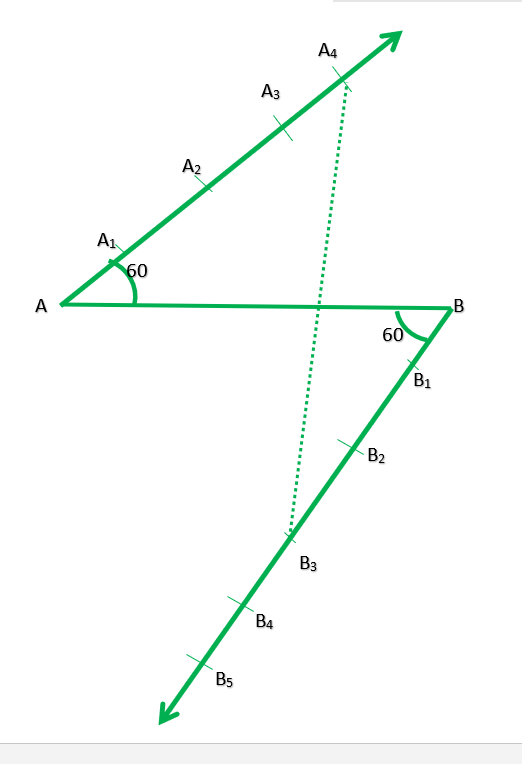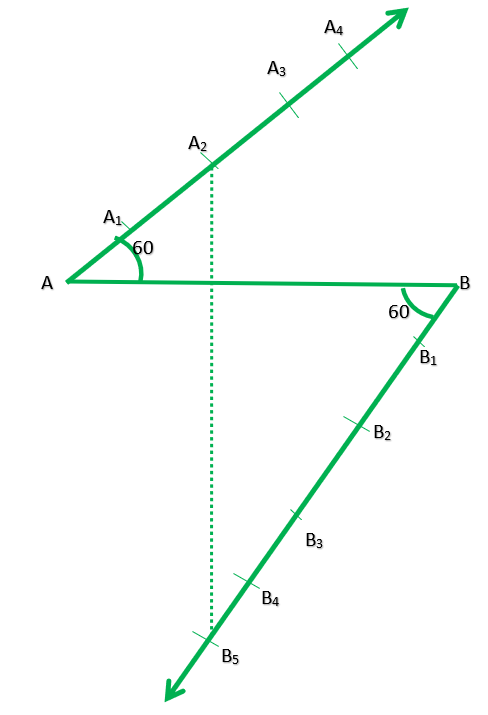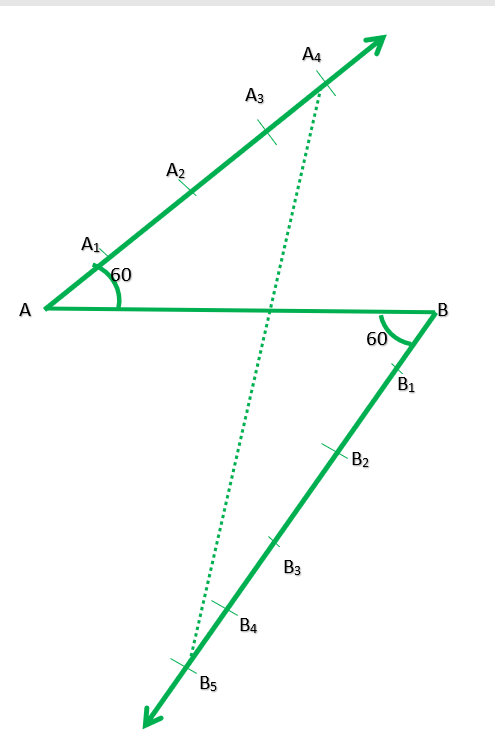GFG App
Open AppBrowser
Continue

# Class 10 RD Sharma Solutions – Chapter 11 Constructions – Exercise 11.1

### Question 1. Determine a point that divides a line segment of length 12 cm internally in the ratio 2 : 3. Also, justify your construction.

Solution:

Steps of construction:

Step 1. Draw a line segment AB =12cm.

Step 2. Through the A and B draw two parallel line on each sides of AB.

Step 3. Cut 2 equal parts on AX and 3 equal parts on BY such that AX1=X1X2  and BX1=Y1Y2=Y2Y3

Step 4. Join X2Y3 which intersects AB at PJustification:

In ∆AX2P and ∆BY3P, we have

∠APX2=∠BPY3          {Because they are vertically opposite angle}

∠X2AP=∠Y3BP

∆AX2P and ∆BY3P {Because AA similarity}

Therefore,{Because of C.P.CT}### Question 2. Divide a line segment of length 9 cm internally in the ratio 4 : 3. Also, give justification of the construction.

Solution:

Steps of construction:

Step 1. Draw a line segment AB = 9 cm.

Step 2. Through the points, A and B, draw two parallel lines AX and BY on the opposite side of AB

Step 3. Cut 4 equal parts on AX and 3 equal parts on BY such AX1=X1X2=X2X3=X3X4 and BY1=Y1Y2=Y2Y3

Step 4. Join x4y3 which intersects AB at P.

Therefore,Justification:

In ∆PX4 and ∆BPY3, we have

∠APX4=∠BPY3         {Because they are vertically opposite angles}

∠PAX4=∠PBY3       {Because they are alternate interior angle}

∆APX4     ∆BPY3   {Because AA similarity}

Therefore,{Because of C.P.C.T}### Question 3. Divide a line segment of length 14 cm internally in the ratio 2 : 5. Also, justify your construction.

Solution:

Steps of construction:

Step 1. Draw a line segment AB = 14 cm.

Step 2. Draw a ray AX making an acute angle with AB.

Step 3. From B, draw another ray BY parallel to AX.

Step 4. From AX, cut off 2 equal parts and from B, cut off 5 equal parts.

Step 5. Join 2 and 5 which intersects AB at P.

P is the required point which divides AB in the ratio of 2 : 5 internally.

Justification:

In ∆PX2 and ∆BPY5, we have

∠APX2=∠BPY5         (vertically opposite angles)

∠PAX2=∠PBY5        (Because they are alternate interior angle)

∆APX    ∆BPY5     (Because AA similarity)

Therefore,(Because of C.P.C.T)### Question 4. Draw a line segment of length 8 cm and divide it internally in the ratio 4 : 5.

Solution:

Steps of construction:

Step 1. Draw a line of 8cm.

Step 2. Through the points, A and B, draw two parallel lines AX and BY on the opposite side of AB

Step 3. Cut 4 equal parts AX and 3 equal parts on BY.

Step 4. Join x4y5 which intersects AB at P.

Justification:

In ∆PX4 and ∆BPY5, we have

∠APX4=∠BPY5         (vertically opposite angles)

∠PAX4=∠PBY5       (alternate interior angle)

∆APX4     ∆BPY5   (AA similarity)

Therefore,(Because of C.P.C.T)My Personal Notes arrow_drop_up